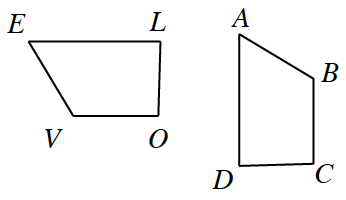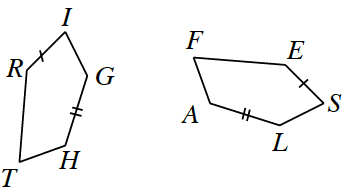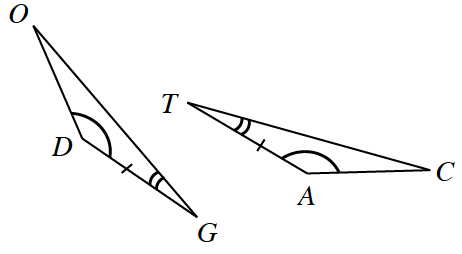Home > INT2 > Chapter 2 > Lesson 2.3.1 > Problem2-82

2-82.

Assume that each pair of figures below is similar. Write a similarity statement to illustrate which parts of each shape correspond. Remember, letter order is important! Homework Help ✎

 a.Hint (a):Which parts of these similar shapes relate to each other?Answer (a):ABCD ~ EVOL b.Hint (b):Use the same method as you did for part (a). c.Hint (c):Name the first triangle in any order. Then determine the order in which the second triangle should be named.